The Sundial Primer created by Carl Sabanski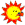The Sundial Primer Index
 Bifilar Sundial Bifilar Sundial: invented in 1922 by Hugo Michnik in its horizontal form, although it can be on any plane. The time is indicated by the intersection on the dial plate, of the shadows of two wires (or other lines in space) stretched above and parallel to it. The wires often run E-W and N-S, with their (different) heights above the plane being a function of of the location of the dial. It may have equiangular hour markings, and hence can be delineated to show many kings of hours. The bifilar sundial can be designed in many variations.  The dial plate and shadow casting wires can be placed in any independent orientation. In fact the wires need not be taut but can be curved if desired.The bifilar sundial must be designed for the particular latitude (ø) where it is to be used. The time is read at the point where the shadows of the two wires intersect. The sundial can be also be used to indicate the date. The sundial can be corrected for longitude and the Equation of Time.The bifilar sundial presented here is in its simplest form. It is designed with equiangular hour lines resulting in the full hour lines being separated by 15º. The two wires are stretched taut, one positioned in the north-south direction and the other in the east west direction. The two wires are 90º apart, i.e. perpendicular to each other. Assume the north-south wire is at a height "hy" above the dial plate. For the hour lines to be equiangular the height "hx" of the east-west wire above the dial plate, and for this particular case the displacement "d" of the east-west wire from the dial centre "C", are determined as follows:hx = hy * sin ød = hy * cos ø Figure 1 shows a drawing of a bifilar sundial designed for a latitude of 50º N. The solstice and equinox declination lines are also shown.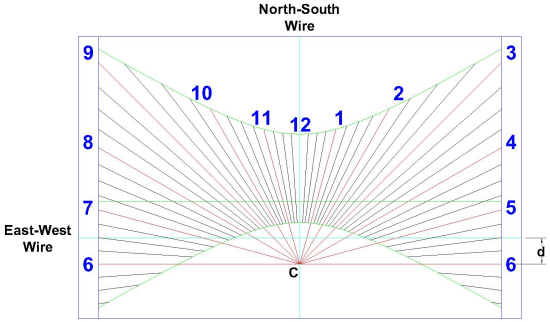Figure 1: Bifilar Sundial (ZW2000/CAD) Because the time is read where the shadows of the two wires intersect care must be taken in determining the height of the two wires above the dial plate. The following figures attempt to illustrate this. The drawings were produced using a bifilar sundial DeltaCad macro written by Valentin Hristov.Figure 2 shows bifilar sundials designed for a latitude of 50º N and dial plates of a fixed size. The difference between the three sundials is the height of the north-south wire "hy". Starting from the top left sundial, the sundial to the right has its wire set twice as high and the one below four times as high.  It can be seen that if the wires are too high the time will be indicated over a shorter period of the year as the intersection point of the shadows moves off the dial plate.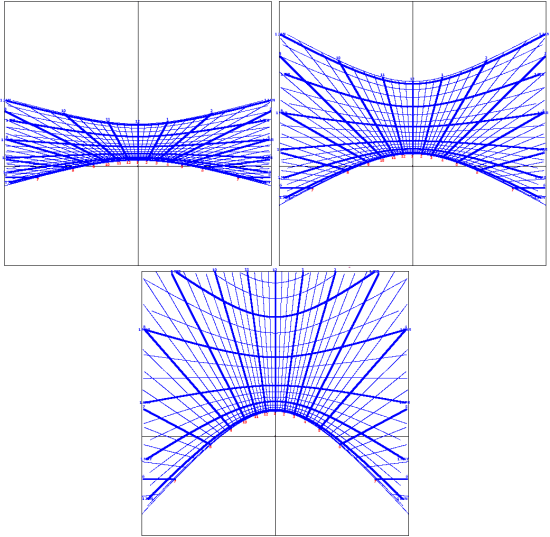Figure 2: Bifilar Sundials - Variable Wire Heights (DELTACAD) Figure 3 shows bifilar sundials designed with a fixed height for the north-south wire "hy" and dial plates of a fixed size. The sundial on the left is designed for a latitude of 30º N and the one on the right for  60º N. Different wire heights can be tried to see what affect they will have. Note that a bifilar sundial at the poles, where hx=hy=1 and d=0, will become an equatorial sundial and one at the Equator, where hx=0, hy=1 and d=1, a polar sundial.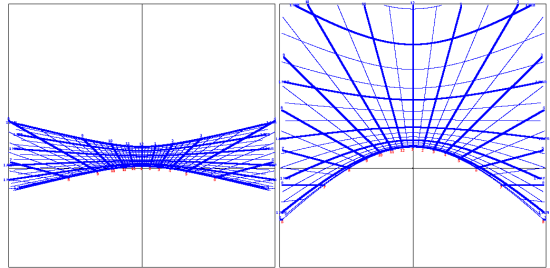Figure 3: Bifilar Sundials - Variable Latitudes (DELTACAD) To determine whether the height of the wires is adequate for a given dial plate size the position of the intersection point of the shadows can be determined at the summer and winter solstices. These two dates will provide the co-ordinates for the two ends of the hour lines. The following assumes the design is of a horizontal bifilar sundial. Think of each wire as a series of points that make up the entire wire. Each point is a nodus of a horizontal sundial and when the position of the shadow cast by that point is found, the shadows of all the other points of that wire will line up. Thus the north-south wire is use to find the "x" co-ordinate of the intersection point and east-west wire is used to find the "y" co-ordinate. The "x" co-ordinate of the intersection point is:x = {hy * cos (DEC) * sin (HLA} / {sin ø * cos (Z - DEC)}The "y" co-ordinate of the intersection point is:y = {hx * cos (DEC) * cos (HLA)} / {sin ø * cos (Z - DEC)}whereZ = arctan {tan ø / cos (h)}and the hour line angles "HLA" for a horizontal sundial can be calculated as follows:HLA = arctan {sin ø * tan (h)} where h is the hour angle, in degrees, given by:h = (T24 - 12) * 15°and T24 is the time in 24-hour clock notation (hours after midnight) in decimal hours.In the above discussion the east west wire is placed a specific distance "d" from the dial centre "C". The critical parameter for establishing  equiangular hour lines is the relationship between "hx" and "hy". The distance "d' can actually be any value.Figure 4 shows bifilar sundials designed for a latitude of 50º N, a fixed height for the north-south wire "hy", a fixed height for the east-west wire "hx" and dial plates of a fixed size. The distance "d" of the east-west wire is being increased but all the sundials are equiangular. However the hour lines are being pushed off the top of the dial plate as "d" increases.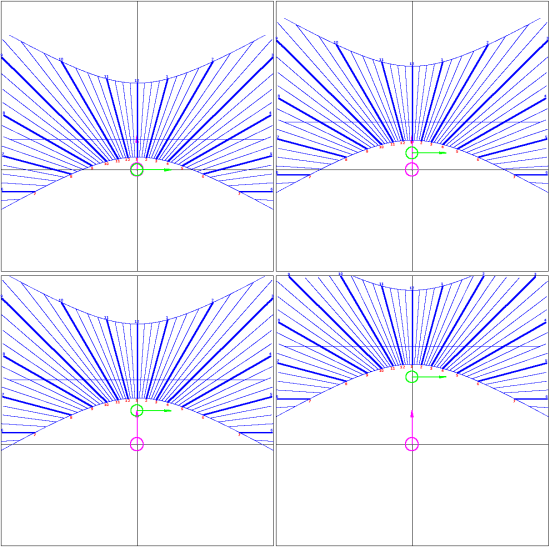Figure 4: Bifilar Sundials - Variable Distance "d" (DELTACAD) When building a bifilar sundial a common way to mount the wires is on posts. The wires must be placed accurately and stretched taut to maximize the accuracy of the sundial.If the east-west posts are designed so they can be rotated to any angle relative to the dial plate the bifilar sundial is then universal. By setting this angle to the latitude then "hx" is equal to "sin (Latitude)" and "d" is equal to "cos (Latitude)". The bifilar sundial shown below is based on the sundial in Figure 1.For an image complete with shadow click here.